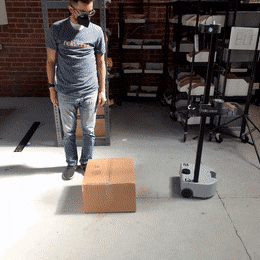# Mobile Base Collision Avoidance

## Example 3

Note

ROS 2 tutorials are still under active development.

The aim of example 3 is to combine the two previous examples and have Stretch utilize its laser scan data to avoid collision with objects as it drives forward.

``````ros2 launch stretch_core stretch_driver.launch.py
``````

Then in a new terminal type the following to activate the LiDAR sensor.

``````ros2 launch stretch_core rplidar.launch.py
``````

To activate the avoider node, type the following in a new terminal.

``````ros2 run stretch_ros_tutorials avoider
``````

To stop the node from sending twist messages, type Ctrl + c in the terminal running the avoider node.### The Code

``````#!/usr/bin/env python3
import rclpy
from rclpy.node import Node
from numpy import linspace, inf, tanh
from math import sin
from geometry_msgs.msg import Twist
from sensor_msgs.msg import LaserScan
class Avoider(Node):
def __init__(self):
super().__init__('stretch_avoider')
self.width = 1
self.extent = self.width / 2.0
self.distance = 0.5
self.twist = Twist()
self.twist.linear.x = 0.0
self.twist.linear.y = 0.0
self.twist.linear.z = 0.0
self.twist.angular.x = 0.0
self.twist.angular.y = 0.0
self.twist.angular.z = 0.0
self.publisher_ = self.create_publisher(Twist, '/stretch/cmd_vel', 1)
self.subscriber_ = self.create_subscription(LaserScan, '/scan', self.set_speed, 10)
def set_speed(self, msg):
angles = linspace(msg.angle_min, msg.angle_max, len(msg.ranges))
points = [r * sin(theta) if (theta < -2.5 or theta > 2.5) else inf for r,theta in zip(msg.ranges, angles)]
new_ranges = [r if abs(y) < self.extent else inf for r,y in zip(msg.ranges, points)]
error = min(new_ranges) - self.distance
self.twist.linear.x = tanh(error) if (error > 0.05 or error < -0.05) else 0
self.publisher_.publish(self.twist)
def main(args=None):
rclpy.init(args=args)
avoider = Avoider()
rclpy.spin(avoider)
avoider.destroy_node()
rclpy.shutdown()
if __name__ == '__main__':
main()
``````

### The Code Explained

Now let's break the code down.

``````#!/usr/bin/env python3
``````

Every Python ROS Node will have this declaration at the top. The first line makes sure your script is executed as a Python script.

``````import rclpy
from rclpy.node import Node
from numpy import linspace, inf, tanh
from math import sin
from geometry_msgs.msg import Twist
from sensor_msgs.msg import LaserScan
``````

You need to import rclpy if you are writing a ROS Node. There are functions from numpy and math that are required within this code, thus linspace, inf, tanh, and sin are imported. The sensor_msgs.msg import is so that we can subscribe to LaserScan messages. The geometry_msgs.msg import is so that we can send velocity commands to the robot.

``````self.publisher_ = self.create_publisher(Twist, '/stretch/cmd_vel', 1)
``````

This declares that your node is publishing to the /stretch/cmd_vel topic using the message type Twist.

``````self.subscriber_ = self.create_subscription(LaserScan, '/scan', self.set_speed, 10)
``````

Set up a subscriber. We're going to subscribe to the topic "/scan", looking for LaserScan messages. When a message comes in, ROS is going to pass it to the function "set_speed" automatically.

``````self.width = 1
self.extent = self.width / 2.0
self.distance = 0.5
``````

self.width is the width of the laser scan we want in front of Stretch. Since Stretch's front is pointing in the x-axis, any points with y coordinates further than half of the defined width (self.extent) from the x-axis are not considered. self.distance defines the stopping distance from an object.

``````self.twist = Twist()
self.twist.linear.x = 0.0
self.twist.linear.y = 0.0
self.twist.linear.z = 0.0
self.twist.angular.x = 0.0
self.twist.angular.y = 0.0
self.twist.angular.z = 0.0
``````

Allocate a Twist to use, and set everything to zero. We're going to do this when the class is initiating. Redefining this within the callback function, `set_speed()` can be more computationally taxing.

``````angles = linspace(msg.angle_min, msg.angle_max, len(msg.ranges))
points = [r * sin(theta) if (theta < -2.5 or theta > 2.5) else inf for r,theta in zip(msg.ranges, angles)]
new_ranges = [r if abs(y) < self.extent else inf for r,y in zip(msg.ranges, points)]
``````

This line of code utilizes linspace to compute each angle of the subscribed scan. Here we compute the y coordinates of the ranges that are below -2.5 and above 2.5 radians of the scan angles. These limits are sufficient for considering scan ranges in front of Stretch, but these values can be altered to your preference. If the absolute value of a point's y-coordinate is under self.extent then keep the range, otherwise use inf, which means "no return".

``````error = min(new_ranges) - self.distance
``````

Calculate the difference of the closest measured scan and where we want the robot to stop. We define this as error.

``````self.twist.linear.x = tanh(error) if (error > 0.05 or error < -0.05) else 0
``````

Set the speed according to a tanh function. This method gives a nice smooth mapping from distance to speed, and asymptotes at +/- 1

``````self.publisher_.publish(self.twist)
``````

Publish the Twist message.

``````def main(args=None):
rclpy.init(args=args)
avoider = Avoider()
rclpy.spin(avoider)
avoider.destroy_node()
rclpy.shutdown()
``````

The next line, rclpy.init() method initializes the node. In this case, your node will take on the name 'stretch_avoider'. NOTE: the name must be a base name, i.e. it cannot contain any slashes "/".

Setup Avoider class with `avoider = Avioder()`

Give control to ROS with `rclpy.spin()`. This will allow the callback to be called whenever new messages come in. If we don't put this line in, then the node will not work, and ROS will not process any messages.